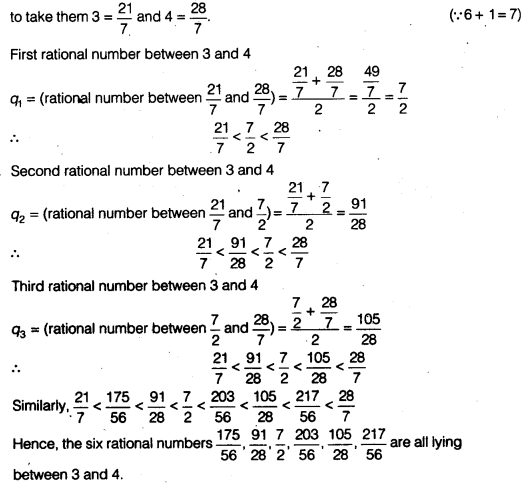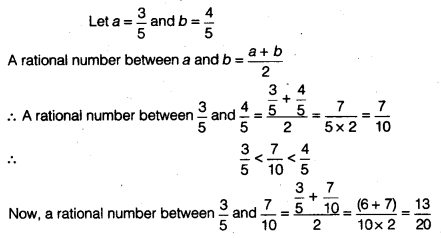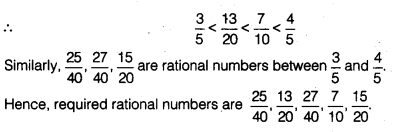# NCERT Solutions for Class 9 Maths Chapter 1 Number Systems Ex 1.1

NCERT Solutions for Class 9 Maths Chapter 1 Number Systems Ex 1.1 are part of Class 9 Maths NCERT Solutions. Here we have given NCERT Solutions for Class 9 Maths Chapter 1 Number Systems Ex 1.1.

 Board CBSE Textbook NCERT Class Class 9 Subject Maths Chapter Chapter 1 Chapter Name Number Systems Exercise Ex 1.1 Number of Questions Solved 4 Category NCERT Solutions

## NCERT Solutions for Class 9 Maths Chapter 1 Number Systems Ex 1.1

Question 1.
Is zero a rational number? Can you write it in the form $$\frac { p }{ q }$$,where p and q are integers and q ≠ 0?
Solution:
Yes, write $$\frac { 0 }{ 1 }$$(where 0 and 1 are integers and q = 1 which is not equal to zero).

Question 2.
Find six rational numbers between 3 and 4.
Solution:
There can be infinitely many rationals between 3 and 4, one way isMore Resources for CBSE Class 9

Question 3.
Find five rational numbers between $$\frac { 3 }{ 5 }$$ and $$\frac { 4 }{ 5 }$$.
Solution:Question 4.
State whether the following statements are true or false. Give reasons for your answers. ‘
(i) Every natural number is a whole number.
(ii) Every integer is a whole number.
(iii) Every rational number is a whole number.
Solution:
(i) True, because natural numbers are 1, 2, 3, 4 ……….∞ and whole
numbers are 0,1, 2, 3, 4, 5, ……..,∞
or
The collection of whole numbers contain all the natural numbers.
(ii) False (∵ negative integers are not included in the list of whole numbers.)
(iii) False [∵ $$\frac { 1 }{ 3 }$$, $$\frac { 6 }{ 7 }$$, $$\frac { 10 }{ 19 }$$ )are not whole numbers.

We hope the NCERT Solutions for Class 9 Maths Chapter 1 Number Systems Ex 1.1, help you. If you have any query regarding NCERT Solutions for Class 9 Maths Chapter 1 Number Systems Ex 1.1, drop a comment below and we will get back to you at the earliest.